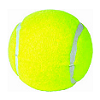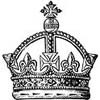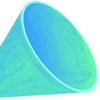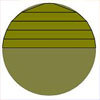Filter by: Content type:
Age range:
Challenge level:

### There are 56 results### Any Win for Tennis?

##### Age 16 to 18 Challenge Level:

What are your chances of winning a game of tennis?### Time to Evolve 2

##### Age 16 to 18 Challenge Level:

How is the length of time between the birth of an animal and the birth of its great great ... great grandparent distributed?### Succession in Randomia

##### Age 16 to 18 Challenge Level:

By tossing a coin one of three princes is chosen to be the next King of Randomia. Does each prince have an equal chance of taking the throne?### Training Schedule

##### Age 14 to 16 Challenge Level:

The heptathlon is an athletics competition consisting of 7 events. Can you make sense of the scoring system in order to advise a heptathlete on the best way to reach her target?### Funnel

##### Age 14 to 16 Challenge Level:

A plastic funnel is used to pour liquids through narrow apertures. What shape funnel would use the least amount of plastic to manufacture for any specific volume ?### Peeling the Apple or the Cone That Lost Its Head

##### Age 14 to 16 Challenge Level:

How much peel does an apple have?### Tree Tops

##### Age 14 to 16 Challenge Level:

Can you make sense of information about trees in order to maximise the profits of a forestry company?### Excel Technique: Triangular Arrays by Turning Off Zeros

##### Age 11 to 16 Challenge Level:

Learn how to use Excel to create triangular arrays.### Excel Interactive Resource: the up and Down Game

##### Age 11 to 16 Challenge Level:

Use an interactive Excel spreadsheet to explore number in this exciting game!### Excel Interactive Resource: Number Grid Functions

##### Age 11 to 16 Challenge Level:

Use Excel to investigate the effect of translations around a number grid.### Excel Investigation: Pascal Multiples

##### Age 11 to 16 Challenge Level:

This spreadsheet highlights multiples of numbers up to 20 in Pascal's triangle. What patterns can you see?### Excel Investigation: Number Pyramids

##### Age 11 to 16 Challenge Level:

Use Excel to create some number pyramids. How are the numbers in the base line related to each other? Investigate using the spreadsheet.### Excel Technique: Conditional Formatting

##### Age 11 to 16 Challenge Level:

Learn how to use conditional formatting to create attractive interactive spreadsheets in Excel.##### Age 14 to 16 Challenge Level:

If a sum invested gains 10% each year how long before it has doubled its value?### Excel Interactive Resource: Interactive Division

##### Age 11 to 16 Challenge Level:

Use an Excel to investigate division. Explore the relationships between the process elements using an interactive spreadsheet.### Take Ten Sticks

##### Age 11 to 16 Challenge Level:

Take ten sticks in heaps any way you like. Make a new heap using one from each of the heaps. By repeating that process could the arrangement 7 - 1 - 1 - 1 ever turn up, except by starting with it?### Excel Investigation: Ring on a String

##### Age 11 to 16 Challenge Level:

This investigation uses Excel to optimise a characteristic of interest.### Euler's Squares

##### Age 14 to 16 Challenge Level:

Euler found four whole numbers such that the sum of any two of the numbers is a perfect square...### Excel Technique: Composite Bar Charts

##### Age 11 to 16 Challenge Level:

Learn how to use composite bar charts in Excel.### Excel Interactive Resource: Equivalent Fraction Bars

##### Age 11 to 16 Challenge Level:

A simple file for the Interactive whiteboard or PC screen, demonstrating equivalent fractions.### Minus One Two Three

##### Age 14 to 16 Challenge Level:

Substitute -1, -2 or -3, into an algebraic expression and you'll get three results. Is it possible to tell in advance which of those three will be the largest ?### Odd Differences

##### Age 14 to 16 Challenge Level:

The diagram illustrates the formula: 1 + 3 + 5 + ... + (2n - 1) = n² Use the diagram to show that any odd number is the difference of two squares.### The Fire-fighter's Car Keys

##### Age 14 to 16 Challenge Level:

A fire-fighter needs to fill a bucket of water from the river and take it to a fire. What is the best point on the river bank for the fire-fighter to fill the bucket ?.### Zeller's Birthday

##### Age 14 to 16 Challenge Level:

What day of the week were you born on? Do you know? Here's a way to find out.### Peaches in General

##### Age 14 to 16 Challenge Level:

It's like 'Peaches Today, Peaches Tomorrow' but interestingly generalized.### Unusual Long Division - Square Roots Before Calculators

##### Age 14 to 16 Challenge Level:

However did we manage before calculators? Is there an efficient way to do a square root if you have to do the work yourself?### Excel Investigation: Happy Numbers

##### Age 11 to 16 Challenge Level:

Take any whole number between 1 and 999, add the squares of the digits to get a new number. Use a spreadsheet to investigate this sequence.### Under the Ribbon

##### Age 14 to 16 Challenge Level:

A ribbon is nailed down with a small amount of slack. What is the largest cube that can pass under the ribbon ?### The Legacy

##### Age 14 to 16 Challenge Level:

Your school has been left a million pounds in the will of an ex- pupil. What model of investment and spending would you use in order to ensure the best return on the money?### There's Always One Isn't There

##### Age 14 to 16 Challenge Level:

Take any pair of numbers, say 9 and 14. Take the larger number, fourteen, and count up in 14s. Then divide each of those values by the 9, and look at the remainders.### Excel Technique: Inserting an Increment Button

##### Age 11 to 16 Challenge Level:

Learn how to use increment buttons and scroll bars to create interactive Excel resources.### Excel Interactive Resource: Fraction Multiplication

##### Age 11 to 16 Challenge Level:

Use Excel to explore multiplication of fractions.##### Age 11 to 16 Challenge Level:

A heap of beads was shared out by a professional bead sharer. Use the information given to find out how many beads there were at the start.### Excel Technique: Making a Table for a Function of Two Independent

##### Age 11 to 16 Challenge Level:

Learn how to make a simple table using Excel.### Excel Interactive Resource: Make a Copy

##### Age 11 to 16 Challenge Level:

Investigate factors and multiples using this interactive Excel spreadsheet. Use the increment buttons for experimentation and feedback.### Negative Power

##### Age 14 to 16 Challenge Level:

What does this number mean ? Which order of 1, 2, 3 and 4 makes the highest value ? Which makes the lowest ?### Pythagoras’ Comma

##### Age 14 to 16 Challenge Level:

Using an understanding that 1:2 and 2:3 were good ratios, start with a length and keep reducing it to 2/3 of itself. Each time that took the length under 1/2 they doubled it to get back within range.### Excel Interactive Resource: Haitch

##### Age 11 to 16 Challenge Level:

An Excel spreadsheet with an investigation.### Excel Investigation: Difference Tuples

##### Age 11 to 16 Challenge Level:

Use an Excel spreadsheet to investigate differences between four numbers. Which set of start numbers give the longest run before becoming 0 0 0 0?### Excel Investigation: Planks

##### Age 11 to 16 Challenge Level:

I have an unlimited supply of planks, of lengths 7 and 9 units. Putting planks end to end, what total lengths can be achieved? Use Excel to investigate.### Excel Investigation: Power Crazy

##### Age 11 to 16 Challenge Level:

When is 7^n + 3^n a multiple of 10? Use Excel to investigate, and try to explain what you find out.### Excel Investigation: the Difference of Two (same) Powers

##### Age 11 to 16 Challenge Level:

If you take two integers and look at the difference between the square of each value, there is a nice relationship between the original numbers and that difference. Can you find the pattern using. . . .### Excel Technique: LOOKUP Functions

##### Age 11 to 16 Challenge Level:

Learn how to use lookup functions to create exciting interactive Excel spreadsheets.### Getting to Know Excel

##### Age 11 to 16 Challenge Level:

A number of useful techniques that can extend your use of Excel.### Excel Investigation: Sums of Consecutive Numbers

##### Age 11 to 16 Challenge Level:### Excel Investigation: Pythagorean Triples

##### Age 11 to 16 Challenge Level:

Use Excel to find sets of three numbers so that the sum of the squares of the first two is equal to the square of the third.### Excel Investigation: Multiples of Three

##### Age 11 to 16 Challenge Level:

Use Excel to investigate digit sums of multiples of three. Can you explain your findings?##### Age 11 to 16 Challenge Level:

Use Excel to investigate remainders and divisors. Was your result predictable?### Excel Technique: LCM & HCF (or GCD)

##### Age 11 to 16 Challenge Level:

Learn how to use the Excel functions LCM and GCD.